•   德国宝沃第二款匠心之作BX5锋芒上市，推出9个版本，售价区间14.98万元-21.68万元。

•   宝沃BX5定位德国劲锐智联SUV， 融合“Young锐年轻动感、Energetic劲活力充沛、Smart智慧非凡”三大产品卖点，为宝沃品牌注入全新活力。

•   宝沃同步出诚意十足的终身免费保修政策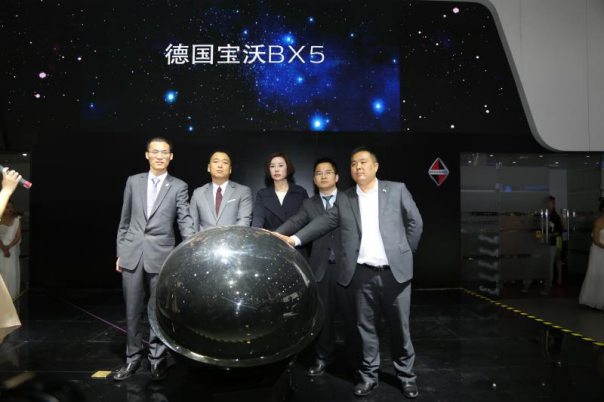劲•锐•智 诠释新德系风范

区别于大家印象中的德系保守形象，BX5秉承宝沃“简单即美”的设计哲学，既可以让人感知到品质和功能的卓越，更可以直击内心、激悦人的灵魂，成为新德系设计的翘楚。宝沃家族的Wingline鹰翼式线条设计DNA，让整车线条犹如疾驰的雄鹰，传达出充沛的力量感;经典的菱形LOGO内嵌于家族式的风叶式八边立体格栅，精致、动感，呈现强烈的视觉张力;1,877mm的同级最宽车身尺寸，加上四轮外扩设计，强化了富于力量感的宽跑姿态。除了有“型”，BX5的座舱设计同样以精致做工、高质感细节和科技感颠覆消费者对SUV内饰粗狂的传统印象，Wingline鹰翼式环体座舱布局、环绕式LED氛围灯、3D高握感三辐方向盘等等，强调座舱整体氛围的同时也兼顾运动基因的表达;悬浮式8英寸中控屏，拥有1,080*720的超高分辨率，让信息更加清晰、易读，也使得座舱的科技感爆棚;通过按60/40比例放倒第二排座椅，最多可实现1230L的超大装载空间，满足居家出行的不同生活需求。

操控和性能从来都是德系品牌的DNA，作为宝沃的重要战略车型，BX5尤其注重操控与驾驶乐趣的表达，以先进的技术造就呼之欲出的澎湃动力和出色的操控体验，激发消费者忠于本心的驾驶欲望

融合宝沃最新科技成果的1.8T涡轮增压直喷发动机，最大功率达到了140 kw，最大扭矩为280 N.m，动力强劲，在同级竞品中几乎无人能敌。而它与爱信6速手自一体变速器的配合，在爆发力与燃油经济性之间找到了完美的结合点。经过纯粹德系调教的前麦弗逊、后多连杆组成的四轮独立悬架系统，不仅可以给车身足够的支撑，还可以将各种“颠簸”拒之门外。博格华纳第五代四驱系统配合 B-Safe智能操控系统(包括CBC、ESC、TCS等16项电子装备),组成同级集成度最高、最智能的电子控制系统，可实现四驱操控、前驱巡航、后驱加速、侧驱过弯、单驱脱困等5大智能控制模式，时刻鼓励你不断探索精准驾控的魅力所在，让每一次愉快的驾驶都安全、安心。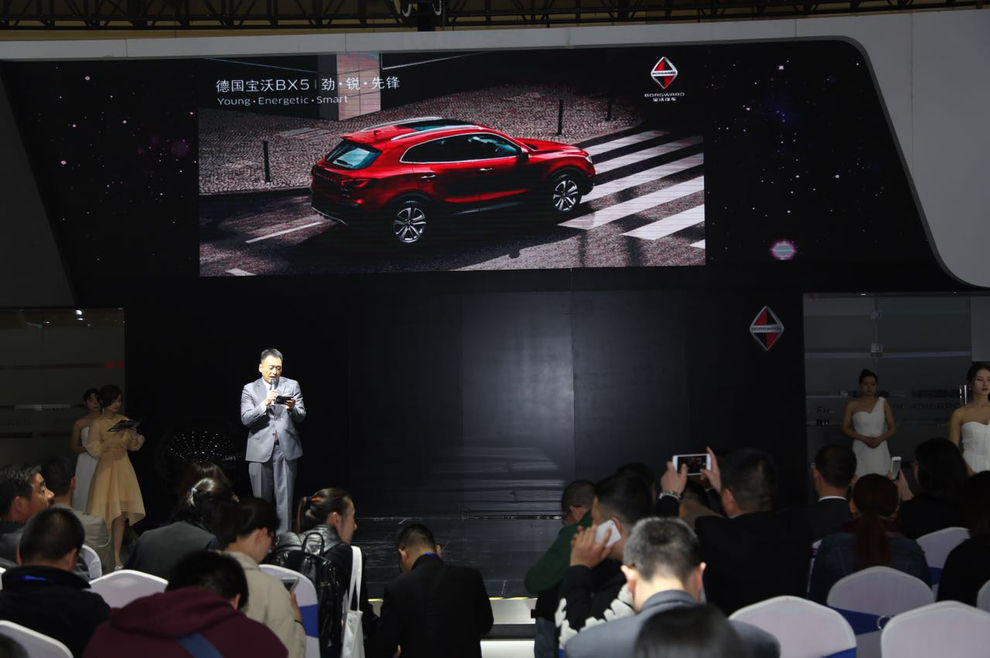宝沃先人一步 树立行业服务新典范

随着消费需求不断升级，消费者愈加关注品质和服务，从而促使汽车业加速向“服务为王”升级转型。在此背景下，宝沃汽车深刻洞悉消费趋势，在赋予旗下车型卓越产品力的同时，更借势强大的品牌精神和实力，相继针对BX7和BX5推出终身免费保修政策，成为市场上第一家敢向消费者承诺“0费用终身保修”的汽车企业，切实保障消费者利益，为客户实现超值驾享体验与服务。宝沃先人一步的政策，极具勇气和诚意，充分彰显宝沃汽车对其产品品质的高度自信。

根据宝沃 “终身免费保修政策”规定，凡BX5首任车主均可享受终身免费保修服务，同时，在整车保修期内还可享受3年免费道路救援的尊享服务※注。这意味着凡购买BX5的消费者，将在宝沃4S店享受终身免费保修，无需支付零部件费用和工时费。尽管易损件不在终身保修范围内，但事实上宝沃对于易损件的保修期也长于其他品牌。目前，在中国市场销售的汽车产品普遍都设定了3-5年的保修期限，超过这个时间，车主就需要自己买单。通过这项服务，用户可以终身享受与新车一样的原厂保修服务，正式开启中国车市“服务为王”的新时代。

BX5的上市，不仅扩大了宝沃SUV家族的阵营，同时，它深植宝沃的血液与基因，前瞻未来，为宝沃品牌注入全新活力，也再次彰显卡尔•宝沃“我要生产汽车”的目标。心动不如行动，消费者可前往宝沃各经销商店预约试驾，体验宝沃BX5。未来，宝沃还将继续以变革精神和创新使命，缔造独具魅力的产品，在未来的出行市场中赢得一席之地，书写新传奇。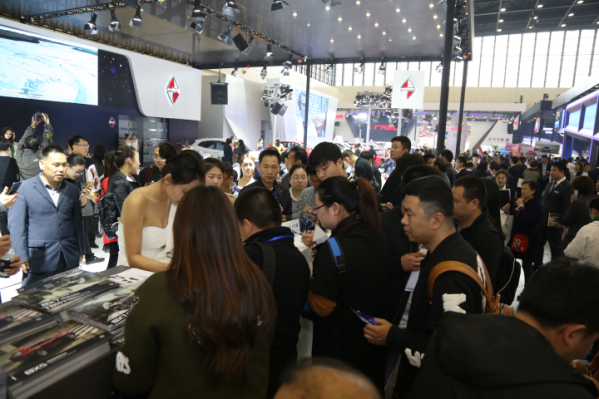※详情请咨询宝沃河南省授权经销商4s店，本活动最终解释权归宝沃汽车（中国）有限公司所有。

BX5官方建议零售价为：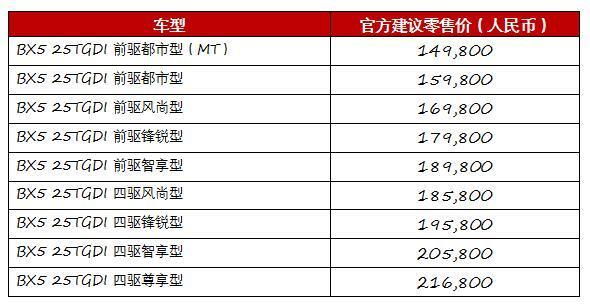- 全文完–

德国宝沃汽车集团简介

传奇百年用荣耀谱写经典

德国BORGWARD(宝沃)汽车集团是由创始人卡尔·宝沃(Carl Borgward)于1919年在德国不来梅创建，其以革命性的技术、全面的产品谱系、超过当时德国60%的出口份额成为德国第三大汽车生产制造商。创新和突破精神是这一扎根不来梅的企业始终坚守的信条。德国BORGWARD(宝沃)汽车打造出了诸多具有划时代意义的轿车及多种交通运输工具，包括轿车、跑车、巴士、消防车、船艇、卡车甚至直升机等。

其中，1949年推出的BORGWARD Hansa 1500作为德国首款采用浮筒式车身设计的轿车，引领了德国汽车造型设计的发展潮流;1954年诞生的BORGWARD Isabella将外观革新、性能优势及燃油经济性集于一身，成为经济奇迹时期的德国梦想之车;1959年上市的BORGWARD P100车型，再次以先驱者的身份，将革命性技术——自动平衡空气弹簧成功应用在汽车制造领域。此外，在1950至1958年间，BORGWARD品牌还积极投身国际赛车领域，Hansa 1500车队在著名的勒芒24小时耐力赛、纽博格林1000公里大赛、卡雷拉泛美拉力赛、法国蒙特赫里大奖赛等多项赛事中取得了骄人成绩，共计打破十二项赛事纪录。

智能驱动以创新引领未来

在经历了充分的探索和准备后，德国BORGWARD (宝沃)汽车集团将秉持“匠心、智能、突破、近享”的品牌价值，致力成为“突破豪华界限的德国智能汽车品牌”，实现“至臻致普智能驱动未来生活”的品牌愿景，以开创性的变革构筑宝沃汽车未来。

德国BORGWARD(宝沃)汽车集团以德国斯图加特为中心，携德国顶级设计师、工程师团队，整合全球资源，以德国扎实精湛的制造工艺，打造稳定而出众的卓越品质;以新能源和智能化，实现经济与社会价值的共赢;以前瞻的思维洞察新时代消费者的需求，打破传统豪华界限，让更多消费者享受到触手可及的豪华体验;以“资源链合，互联共享”的全新模式，推动全球化布局，为全球汽车用户带来超越期待的完美驾乘体验，缔造智能化用车生活生态圈。

德国BORGWARD(宝沃)汽车集团于2015年法兰克福车展发布了首款定位为德国宽体智联SUV车型BX7及BX7 TS。BX7定位德国宽体智联SUV，于2016年正式登陆中国市场，截至目前已取得超过4万台销售订单。同年日内瓦车展上，定位于中高端紧凑型SUV的全新BX5概念车和定位于豪华运动型SUV的BX6 TS概念车亦惊艳亮相。作为宝沃汽车第二款创新力作，BX5定位德国劲锐智联SUV，于2017年3月24日正式上市。自此，德国BORGWARD(宝沃)汽车集团SUV产品谱系雏形初现。宝沃倾力打造的全新产品将严谨工艺、先进技术与鲜明个性完美结合，将为消费者提供德系SUV车型全新选择。|分享

•已阅！握爪
•我手滑为你点赞
•128个赞！
•不明觉厉
•阅后既醉
•有钱！任性
•照片太美，我不敢看
•干货！杠杠的
•高大上
•膜拜中！
•请接受我的膝盖
•猫哥/猫妹么么哒
•神吐槽
•我只笑笑不说话
•我想静静
•窒息
##### 资讯相关车系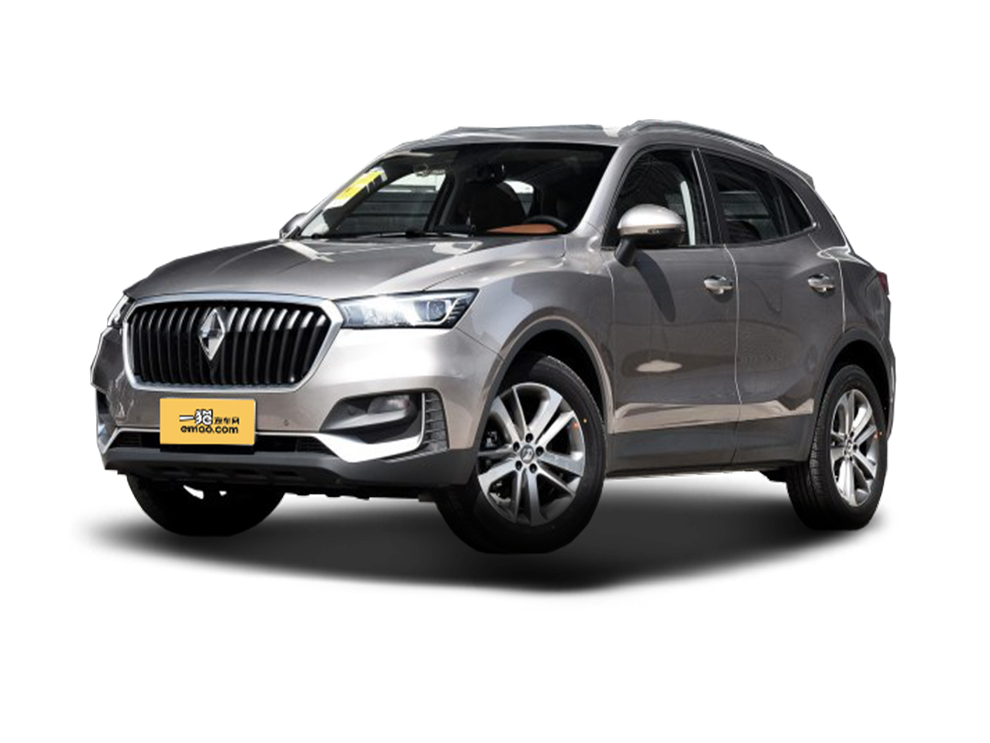##### 热度排行• 上汽斯柯达

• 阿斯顿·马丁
• 奥迪
• 阿尔法罗密欧
• ALPINA

• 巴博斯
• 宝骏
• 宝马
• 保时捷
• 北汽制造
• 奔驰
• 奔腾
• 本田
• 比亚迪
• 标致
• 别克
• 宾利
• 布加迪
• 北汽威旺
• 北京
• 北汽绅宝
• 北汽幻速
• 北汽新能源
• 宝沃
• 比速汽车
• 北汽道达
• 北京现代

• 昌河
• 长安
• 长城
• 长安商用
• 成功汽车
• 长江EV
• 长安轻车型

• 大众
• 道奇
• 东风
• 东风风神
• 东风小康
• 东南
• DS
• 东风风行
• 东风风度
• 东风风光
• 东风启辰
• 东风日产

• 法拉利
• 菲亚特
• 丰田
• 福迪
• 福特
• 福田汽车
• 福汽启腾
• 风诺

• GMC
• 广汽传祺
• 广汽吉奥
• 观致

• 哈飞
• 海格
• 海马
• 华泰
• 黄海
• 恒天
• 红旗
• 哈弗
• 华颂
• 华凯
• 华泰新能源
• 汉腾汽车

• Jeep
• 江淮
• 江铃
• 捷豹
• 金杯
• 九龙
• 吉利汽车
• 金旅
• 金龙
• 江铃集团轻汽
• 江铃集团新能源
• 君马汽车
• 捷途

• 开瑞
• 凯迪拉克
• 科尼赛克
• 克莱斯勒
• KTM
• 卡威
• 凯翼
• 康迪
• 康迪电动汽车集团

• 猎豹汽车
• 兰博基尼
• 劳斯莱斯
• 雷克萨斯
• 雷诺
• 理念
• 力帆
• 莲花汽车
• 林肯
• 铃木
• 陆风
• 路虎
• 路特斯
• 领志
• 领克
• 零跑汽车

• MG
• MINI
• 马自达
• 玛莎拉蒂
• 迈凯伦
• 摩根

• 纳智捷

• 讴歌
• 欧宝
• 欧朗
• 欧拉
• 欧尚汽车

• 帕加尼

• 奇瑞
• 启辰
• 起亚
• 前途
• 庆铃汽车

• 日产
• 荣威
• 瑞麒汽车
• 如虎
• 瑞驰

• 上汽大通
• smart
• 三菱
• 双环
• 双龙
• 斯巴鲁
• 斯柯达
• 萨博
• 思铭
• 赛麟
• SWM斯威汽车
• 上汽大众

• TESLA
• 泰卡特
• 腾势

• 威麟
• 威兹曼
• 沃尔沃
• 五菱汽车
• 五十铃
• 潍柴英致
• WEY
• 蔚来
• 威马汽车

• 现代
• 雪佛兰
• 雪铁龙
• 西雅特
• 新特汽车
• 小鹏汽车
• 新宝骏

• 野马汽车
• 一汽
• 依维柯
• 英菲尼迪
• 永源
• 驭胜

• 中华
• 中兴
• 众泰
• 知豆
• 之诺
• 正道汽车
• A
• B
• C
• D
• E
• F
• G
• H
• I
• J
• K
• L
• M
• N
• O
• P
• Q
• R
• S
• T
• U
• V
• W
• X
• Y
• Z

• 上汽斯柯达

• 阿斯顿·马丁
• 奥迪
• 阿尔法罗密欧
• ALPINA

• 巴博斯
• 宝骏
• 宝马
• 保时捷
• 北汽制造
• 奔驰
• 奔腾
• 本田
• 比亚迪
• 标致
• 别克
• 宾利
• 布加迪
• 北汽威旺
• 北京
• 北汽绅宝
• 北汽幻速
• 北汽新能源
• 宝沃
• 比速汽车
• 北汽道达
• 北京现代

• 昌河
• 长安
• 长城
• 长安商用
• 成功汽车
• 长江EV
• 长安轻车型

• 大众
• 道奇
• 东风
• 东风风神
• 东风小康
• 东南
• DS
• 东风风行
• 东风风度
• 东风风光
• 东风启辰
• 东风日产

• 法拉利
• 菲亚特
• 丰田
• 福迪
• 福特
• 福田汽车
• 福汽启腾
• 风诺

• GMC
• 广汽传祺
• 广汽吉奥
• 观致

• 哈飞
• 海格
• 海马
• 华泰
• 黄海
• 恒天
• 红旗
• 哈弗
• 华颂
• 华凯
• 华泰新能源
• 汉腾汽车

• Jeep
• 江淮
• 江铃
• 捷豹
• 金杯
• 九龙
• 吉利汽车
• 金旅
• 金龙
• 江铃集团轻汽
• 江铃集团新能源
• 君马汽车
• 捷途

• 开瑞
• 凯迪拉克
• 科尼赛克
• 克莱斯勒
• KTM
• 卡威
• 凯翼
• 康迪
• 康迪电动汽车集团

• 猎豹汽车
• 兰博基尼
• 劳斯莱斯
• 雷克萨斯
• 雷诺
• 理念
• 力帆
• 莲花汽车
• 林肯
• 铃木
• 陆风
• 路虎
• 路特斯
• 领志
• 领克
• 零跑汽车

• MG
• MINI
• 马自达
• 玛莎拉蒂
• 迈凯伦
• 摩根

• 纳智捷

• 讴歌
• 欧宝
• 欧朗
• 欧拉
• 欧尚汽车

• 帕加尼

• 奇瑞
• 启辰
• 起亚
• 前途
• 庆铃汽车

• 日产
• 荣威
• 瑞麒汽车
• 如虎
• 瑞驰

• 上汽大通
• smart
• 三菱
• 双环
• 双龙
• 斯巴鲁
• 斯柯达
• 萨博
• 思铭
• 赛麟
• SWM斯威汽车
• 上汽大众

• TESLA
• 泰卡特
• 腾势

• 威麟
• 威兹曼
• 沃尔沃
• 五菱汽车
• 五十铃
• 潍柴英致
• WEY
• 蔚来
• 威马汽车

• 现代
• 雪佛兰
• 雪铁龙
• 西雅特
• 新特汽车
• 小鹏汽车
• 新宝骏

• 野马汽车
• 一汽
• 依维柯
• 英菲尼迪
• 永源
• 驭胜

• 中华
• 中兴
• 众泰
• 知豆
• 之诺
• 正道汽车
• A
• B
• C
• D
• E
• F
• G
• H
• I
• J
• K
• L
• M
• N
• O
• P
• Q
• R
• S
• T
• U
• V
• W
• X
• Y
• Z

• 上汽斯柯达

• 阿斯顿·马丁
• 奥迪
• 阿尔法罗密欧
• ALPINA

• 巴博斯
• 宝骏
• 宝马
• 保时捷
• 北汽制造
• 奔驰
• 奔腾
• 本田
• 比亚迪
• 标致
• 别克
• 宾利
• 布加迪
• 北汽威旺
• 北京
• 北汽绅宝
• 北汽幻速
• 北汽新能源
• 宝沃
• 比速汽车
• 北汽道达
• 北京现代

• 昌河
• 长安
• 长城
• 长安商用
• 成功汽车
• 长江EV
• 长安轻车型

• 大众
• 道奇
• 东风
• 东风风神
• 东风小康
• 东南
• DS
• 东风风行
• 东风风度
• 东风风光
• 东风启辰
• 东风日产

• 法拉利
• 菲亚特
• 丰田
• 福迪
• 福特
• 福田汽车
• 福汽启腾
• 风诺

• GMC
• 广汽传祺
• 广汽吉奥
• 观致

• 哈飞
• 海格
• 海马
• 华泰
• 黄海
• 恒天
• 红旗
• 哈弗
• 华颂
• 华凯
• 华泰新能源
• 汉腾汽车

• Jeep
• 江淮
• 江铃
• 捷豹
• 金杯
• 九龙
• 吉利汽车
• 金旅
• 金龙
• 江铃集团轻汽
• 江铃集团新能源
• 君马汽车
• 捷途

• 开瑞
• 凯迪拉克
• 科尼赛克
• 克莱斯勒
• KTM
• 卡威
• 凯翼
• 康迪
• 康迪电动汽车集团

• 猎豹汽车
• 兰博基尼
• 劳斯莱斯
• 雷克萨斯
• 雷诺
• 理念
• 力帆
• 莲花汽车
• 林肯
• 铃木
• 陆风
• 路虎
• 路特斯
• 领志
• 领克
• 零跑汽车

• MG
• MINI
• 马自达
• 玛莎拉蒂
• 迈凯伦
• 摩根

• 纳智捷

• 讴歌
• 欧宝
• 欧朗
• 欧拉
• 欧尚汽车

• 帕加尼

• 奇瑞
• 启辰
• 起亚
• 前途
• 庆铃汽车

• 日产
• 荣威
• 瑞麒汽车
• 如虎
• 瑞驰

• 上汽大通
• smart
• 三菱
• 双环
• 双龙
• 斯巴鲁
• 斯柯达
• 萨博
• 思铭
• 赛麟
• SWM斯威汽车
• 上汽大众

• TESLA
• 泰卡特
• 腾势

• 威麟
• 威兹曼
• 沃尔沃
• 五菱汽车
• 五十铃
• 潍柴英致
• WEY
• 蔚来
• 威马汽车

• 现代
• 雪佛兰
• 雪铁龙
• 西雅特
• 新特汽车
• 小鹏汽车
• 新宝骏

• 野马汽车
• 一汽
• 依维柯
• 英菲尼迪
• 永源
• 驭胜

• 中华
• 中兴
• 众泰
• 知豆
• 之诺
• 正道汽车
• A
• B
• C
• D
• E
• F
• G
• H
• I
• J
• K
• L
• M
• N
• O
• P
• Q
• R
• S
• T
• U
• V
• W
• X
• Y
• Z